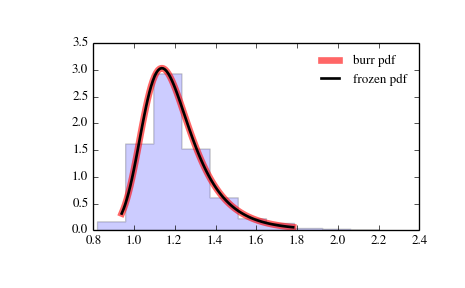# scipy.stats.burr¶

scipy.stats.burr = <scipy.stats._continuous_distns.burr_gen object at 0x2b45d2fa1910>[source]

A Burr continuous random variable.

Continuous random variables are defined from a standard form and may require some shape parameters to complete its specification. Any optional keyword parameters can be passed to the methods of the RV object as given below:

Parameters: x : array_like quantiles q : array_like lower or upper tail probability c, d : array_like shape parameters loc : array_like, optional location parameter (default=0) scale : array_like, optional scale parameter (default=1) size : int or tuple of ints, optional shape of random variates (default computed from input arguments ) moments : str, optional composed of letters [‘mvsk’] specifying which moments to compute where ‘m’ = mean, ‘v’ = variance, ‘s’ = (Fisher’s) skew and ‘k’ = (Fisher’s) kurtosis. (default=’mv’) Alternatively, the object may be called (as a function) to fix the shape, location, and scale parameters returning a “frozen” continuous RV object: rv = burr(c, d, loc=0, scale=1) Frozen RV object with the same methods but holding the given shape, location, and scale fixed.

fisk
a special case of burr with d = 1

Notes

The probability density function for burr is:

```burr.pdf(x, c, d) = c * d * x**(-c-1) * (1+x**(-c))**(-d-1)
```

for x > 0.

Examples

```>>> from scipy.stats import burr
>>> import matplotlib.pyplot as plt
>>> fig, ax = plt.subplots(1, 1)
```

Calculate a few first moments:

```>>> c, d = 10.5, 4.3
>>> mean, var, skew, kurt = burr.stats(c, d, moments='mvsk')
```

Display the probability density function (pdf):

```>>> x = np.linspace(burr.ppf(0.01, c, d),
...               burr.ppf(0.99, c, d), 100)
>>> ax.plot(x, burr.pdf(x, c, d),
...          'r-', lw=5, alpha=0.6, label='burr pdf')
```

Alternatively, freeze the distribution and display the frozen pdf:

```>>> rv = burr(c, d)
>>> ax.plot(x, rv.pdf(x), 'k-', lw=2, label='frozen pdf')
```

Check accuracy of cdf and ppf:

```>>> vals = burr.ppf([0.001, 0.5, 0.999], c, d)
>>> np.allclose([0.001, 0.5, 0.999], burr.cdf(vals, c, d))
True
```

Generate random numbers:

```>>> r = burr.rvs(c, d, size=1000)
```

And compare the histogram:

```>>> ax.hist(r, normed=True, histtype='stepfilled', alpha=0.2)
>>> ax.legend(loc='best', frameon=False)
>>> plt.show()
```Methods

 rvs(c, d, loc=0, scale=1, size=1) Random variates. pdf(x, c, d, loc=0, scale=1) Probability density function. logpdf(x, c, d, loc=0, scale=1) Log of the probability density function. cdf(x, c, d, loc=0, scale=1) Cumulative density function. logcdf(x, c, d, loc=0, scale=1) Log of the cumulative density function. sf(x, c, d, loc=0, scale=1) Survival function (1-cdf — sometimes more accurate). logsf(x, c, d, loc=0, scale=1) Log of the survival function. ppf(q, c, d, loc=0, scale=1) Percent point function (inverse of cdf — percentiles). isf(q, c, d, loc=0, scale=1) Inverse survival function (inverse of sf). moment(n, c, d, loc=0, scale=1) Non-central moment of order n stats(c, d, loc=0, scale=1, moments=’mv’) Mean(‘m’), variance(‘v’), skew(‘s’), and/or kurtosis(‘k’). entropy(c, d, loc=0, scale=1) (Differential) entropy of the RV. fit(data, c, d, loc=0, scale=1) Parameter estimates for generic data. expect(func, c, d, loc=0, scale=1, lb=None, ub=None, conditional=False, **kwds) Expected value of a function (of one argument) with respect to the distribution. median(c, d, loc=0, scale=1) Median of the distribution. mean(c, d, loc=0, scale=1) Mean of the distribution. var(c, d, loc=0, scale=1) Variance of the distribution. std(c, d, loc=0, scale=1) Standard deviation of the distribution. interval(alpha, c, d, loc=0, scale=1) Endpoints of the range that contains alpha percent of the distribution